Mobile QR Code1. (Smart-City Service Group Hyundai-Autoever / 510 Teheran-ro, Gangnam-gu, Korea {dg.ko, hlcho}@hyundai-autoever.com )
2. (Smart-Factory of Next-Generation Service Group, Hyundai-Autoever / 510 Teheran-ro, Gangnam-gu, Korea ymyoon@hyndai-autover.com )
3. (Advanced Systems Convergence Lab, Department of Systems Engineering, Graduate School, Ajou University / 206 World cup-ro Yeongtong-gu, Suwon-si, Gyeonggi-do, Korea jokim1201@coredit.co.kr,       CEO of Core DIT Co.,Ltd / 21, Bangbaecheon-ro 2-gil, Seocho-gu, Seoul, Korea jokim1201@coredit.co.kr )

LSTM, CNN, Electricity demand prediction, Deep-learning, Machine-learning, ARIMA, MLP

## 1. Introduction

An electricity demand prediction system is an important factor for an energy management system for capacity planning and maintenance scheduling for power control systems. For this reason, electricity demand prediction system has been widely challenged a lot of filed [1, 2, 13, 14]. Recently, many electric vehicles (EVs) have been emerging instead of internal combustion engine vehicles. Along with this, an energy management system (EMS) is beginning to gain interest using EVs for efficient charging and discharging with optimized planning. Therefore, an electricity demand prediction system has an important role in reducing energy wastage and making optimal planning.

Usually, electricity demand prediction is divided into three levels: long term, medium term. and short term. In this paper, we target the short term and have built a dataset for 1 year (365 days, 7,760 hours) of training data with about 25 days (60 hours) of validation data from a German house. The goal of this work is to analyze the performance of ARIMA, eMLP, and CNN-LSTM models by MSE and MAPE with a dataset that has a non-uniform electricity consumption pattern. From this, we will be able to decrease energy wastage. Our main idea is to apply an optimal plan for EV-V1G, V2G/L, and an Energy Storage System (ESS). We can protect and save energy when there is reverse power flow periodically if we can predict electricity demand well in a PV or ESS environment.

The rest of this paper is organized as follows. In the next section, we describe the dataset for experiments. Section 3 briefly overviews models for performance comparison, and we delineate a CNN-LSTM method. In section 4, we report the experimental results for ARIMA, eMLP, and CNN-LSTM. We also give an analysis of the performance comparison for the mean square error (MSE) and MAPE. Finally, in section 5, we present a conclusion and suggest future work.

## 2. Related Work

Electricity demand prediction system has been challenging among researchers over the past few decades, according to Taylor $\textit{et al.}$  compared electricity demand prediction by the mean absolute percentage error (MAPE) using the autoregressive moving average (ARMA) and principal component analysis (PCA) and evaluated them by European electricity demand data. However, recently, time-series prediction system tasks exploit machine learning and deep learning technologies .

To predict electricity demand, autoregressive integrated moving average (ARIMA) and sequence-to-sequence long short-term memory (S2S-LSTM) models have been used often to solve this problem. In terms of performance, it showed reasonable results. In addition, according to Fan $\textit{et al.}$  enhanced electricity demand prediction methods such as K-means and the Pearson correlation coefficient to add human behavior patterns. Artificial neural networks (ANNs) have shown efficient performance in a prediction system. However, one study  showed that an ANN is not proper for predicting electricity demand.

Multi-layer perceptron (MLP) is the most popular method to predict electricity demand . We have designed a method using k-means with MLP, and we added an error correction routine to enhance accuracy using the last 15 days of ground truth with predicted values. We called this model error-corrected MLP (eMLP).

## 3. Methodologies

In this section, we describe electricity demand prediction methods.

### 3.1 eMLP

The MLP model has been a widely used methodology to predict electricity demand. In many studies [3, 8, 15], the performance has already been verified. Therefore, we have designed the MLP part as shown in Fig. 1.

To increase accuracy, we added an error correction method to the output of the PSO, which is an optimized weighted summation between MLP and k-means. We named this model eMLP. For this model, we have tried to increase the accuracy using k-means clustering, GaussianNB, and Ensemble  with PSO, as in Fig. 1(c).

The input features are the weather description, day of the week, and a holiday or weekday. For this, we process binary data as "1" or "0". An example of weather description is if the weather is "clear", the input vector is expressed by "0 0 0 1". "Cloud" is "0 0 1 0", "rain or snow" is "0 1 0 0", and "sunny" is "1 0 0 0". Additionally, the day of the week is also described in binary, such as "1 0 0 0 0 0 0" for Monday and "0 0 0 0 0 0 1" for Sunday. Finally, the eMLP model procedure is as follows:

 a. Input dimension is 14 (weather forecast with maximum temperature, day of the week, holiday) b. Processing 5-layers MLP (hidden size is 20, 20, 20, 20, 15) as in Fig. 3(a) c. Conducting k-means clustering using past 1 year of actual electricity demand data and optimization of "k" using the error (between actual data and predicted data) d. Processing GaussianNB for each cluster and finding weighting value (build a model for each cluster) e. Weighted summation using the output of step "b" and step "d" f. More processing for MLP, which is error correction, which consists of 4 layers (20, 20, 20, 15) and finding predicted electricity demand, as in Fig. 3(b)
##### Fig. 1. The architecture of eMLP model: (a) the architecture of MLP, (b) error correction design, (c) the data flow of eMLP model.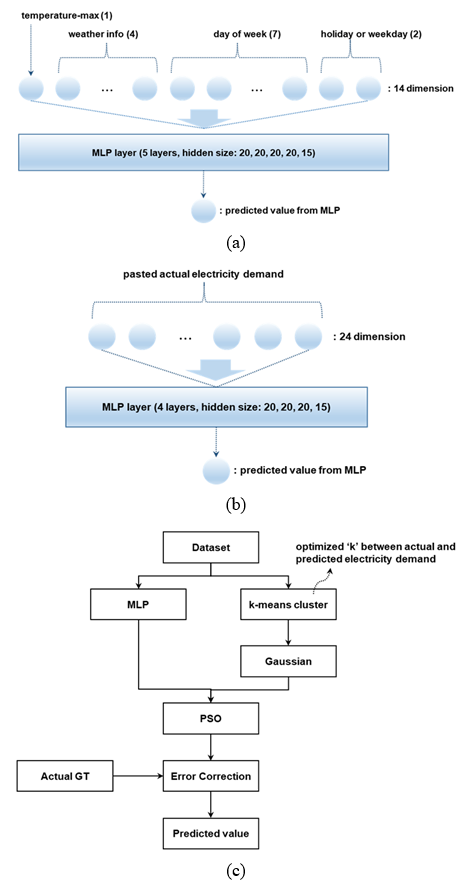### 3.2 ARIMA

ARIMA [21-23] is a generalization of the auto-regressive moving average model and mainly used for time-series analysis. The ARIMA model elements are as follows :

 $\textit{AR}$: It has a meaning of "Auto-regression" and is a regression model between observation and a number of lagged observations ($\textit{p}$) $\textit{I}$: It means "Integrated" and is used to make the time series stationary, which measures different times ($\textit{d}$) $\textit{MA}$: "Moving Average" is an approach that can consider the dependency between observed samples and the residual error terms when a moving average model is used with a number of lagged observations ($\textit{q}$)

AR is written as a linear regression (Eq. (1)):

##### (1)
$x_{t}=b+\sum _{t=1}^{p}\,\varnothing _{i}\,x_{t-i}+\varepsilon _{t}$

where $x_{t}$ is the stationary variable value at time t. $\varnothing _{i}$ is the autocorrelation coefficient is estimated by lags $\textit{1}$ to $p\,.$ Lastly, $\varepsilon _{t}$ is the residual. The MA model of $\textit{q}$, MA($\textit{q}$), is written as below (Eq. (2)):

##### (2)
$x_{t}=\mu +\sum _{t=1}^{q}\theta _{t}\varepsilon _{t-i}$

where $\mu$ is the expected $x_{t}$, and $\theta _{t}$ is the coefficient to be estimated. The ARIMA model for order ($\textit{p}$, $\textit{0}$, $\textit{q}$) is calculated as below (Eq. (3)):

##### (3)
$x_{t}=b+\sum _{i=1}^{p}\varnothing _{i=1}x_{t-i}+\varepsilon _{t}+\sum _{i=1}^{q}\theta _{i}\varepsilon _{t-i}$

In this work, we used optimized values: $\textit{p}$ is 4, $\textit{d}$ is 0: and $\textit{q}$ is 2. These values reached the best MSE and MAPE.

### 3.3 CNN-LSTM

We designed CNN-LSTM [24,25], and our goal was obviously to have higher performance. This approach is inspired by convolutional neural networks (CNN) and long short-term memory (LSTM). This model requires hourly weather forecast data and day information (what day of the week it is, whether it is a holiday, and so on) and the information of the weather forecast for an input vector of the network structure, as shown in Fig. 1. To predict the next hour, weather forecast information with day information for the past 6 hours is required, such as humidity, temperature, weather information, month, day, holiday, and day of the week for each hour. After building an 84-dimensional input vector, we generate a 40-dimensional vector by 4 layers of a simple multi-layer neural network, as in Fig. 2(a).

For this input vector, actual electricity demand for the past 24 hours is additionally inserted, as in Fig. 2(b). The next step is building an embedding vector for weatherID, which proceeds with a CNN. It also is used to predict the required past 6 hours weatherID information and has a value range with indexing , as in Table 1. The embedding vector of weatherID has been used to configure the convolutional network, as in Fig. 3.

The weatherID is converted into a 30-dimensional embedding vector. Therefore, a 6 x 30 matrix can be created by composing it from the past 6 hours. After the 1D-CNN and max-pooling process, the final output becomes 30-dimensional and is concatenated with the output of Fig. 4(a). Finally, to predict the electricity demand value, a bi-directional LSTM network was designed, as shown in Fig. 4, and the total process is as follows:

 a. Generating a 40-dimensional input from 4 layers of a multi-neural network, which consists of a rectified linear unit activation function (using past 6 hours of data with past 6 to 30 hours of actual electricity demand value) b. Converting a weatherID to 30-dimensional embedding vector (we created a 6 x 30 matrix; for this reason, we used past 6 hours of data) c. Processing 1D-CNN (size is 3x1, filter is 30, activation function is rectified linear unit function, padding method is "fill with the same value") d. Processing 6x1 max-pooling (output matrix is 1 x 30, flatten 30-dimension output) e. Concatenating output values of step "a" and step "d" (created 70-dimension vectors for input of LSTM processing) f. Processing bi-directional LSTM (hidden layer consists of only one, hidden size is 100, and activation function is tanh) g. Finally, a dense layer is used to find a predicted electricity demand value from the hidden output of bi-directional LSTM layer
##### Table 1. Information of input for CNN (weather ID which is re-mapped, has 28 range).
 ID Description 1 thunderstorm with rain 2 light intensity drizzle 3 drizzle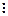20 mist 21 haze28 overcast clouds: 85 - 100%
##### Fig. 2. The structure of weather forecast for input vector (before concatenating): (a) the neural network with input data, (b) the structure of input information.##### Fig. 3. CNN structure before concatenating.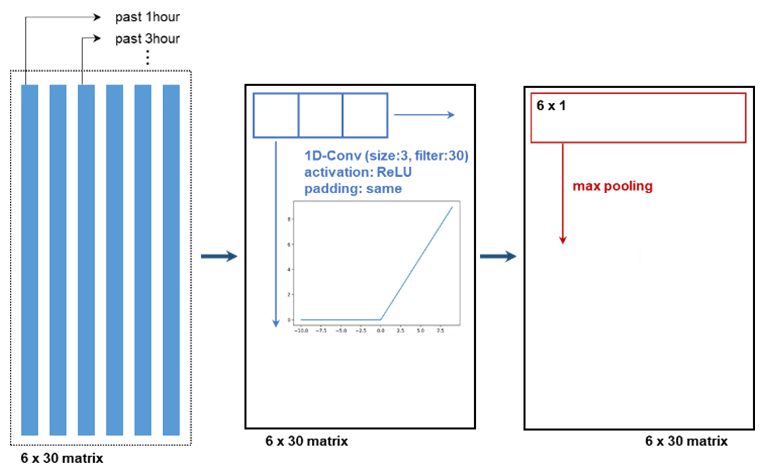##### Fig. 4. The LSTM structure of finding predicted electricity demand.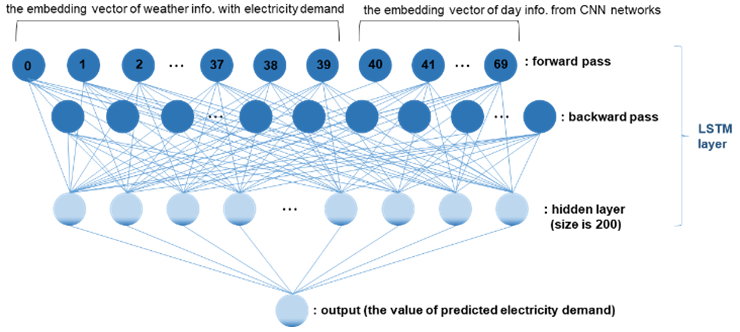##### Fig. 5. An electricity demand dataset that has a regular pattern: (a) weekday, (b) weekend including holiday.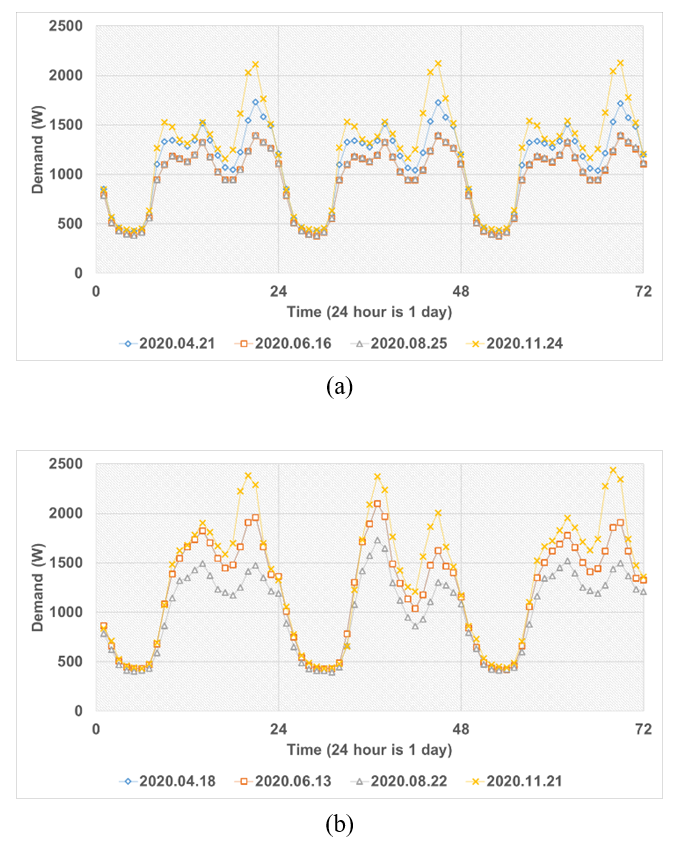##### Fig. 6. An electricity demand dataset that does not have a pattern: (a) weekday, (b) weekend including holiday.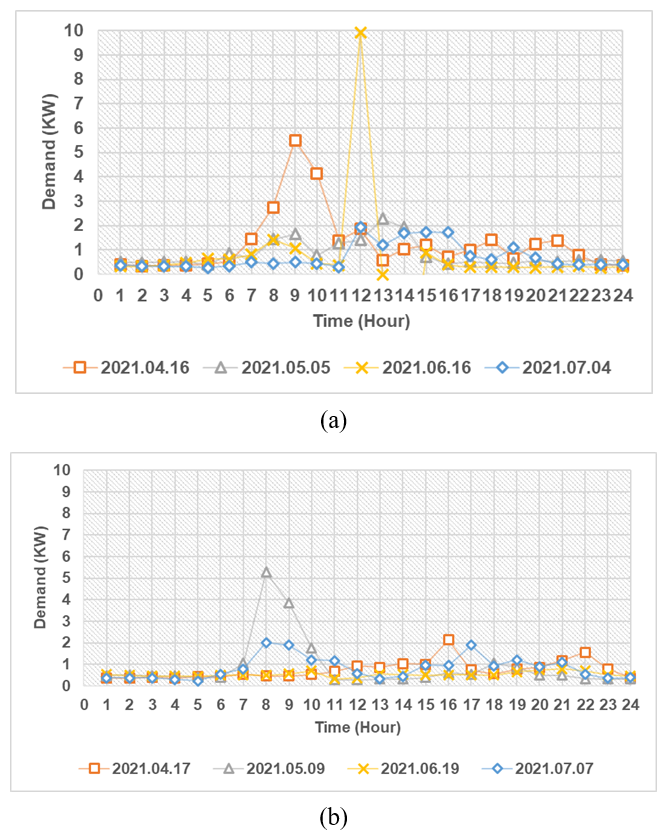## 4. Performance Evaluation

### 4.1 Dataset

To predict electricity demand, many features of weather forecasts and weather information are required, such as temperature, humidity, wind speed, precipitation, snowfall, day of the week, weather description, and holiday information. In addition, the task which predicts the electricity usage of a household has regular patterns that are relatively simple, as shown in Fig. 5. However, for this work, we collected and built a dataset from a household that does not have an electricity usage pattern, as shown in Fig. 6. In the case of this household, unlike a South Korean household, it has a different electricity usage pattern on each day.

In Fig. 5, the dataset was extracted from a South Korean household, and in Fig. 6, the dataset was extracted from a German household. Fig. 5 shows a general pattern for each hour. Electricity usage is increasing in the evening when people are staying at home, and electricity usage is low in the afternoon when people are going out. This electricity usage pattern can be easily solved. However, in the case of Fig. 6, to solve this problem, we need many features, such as past electricity usage, weather forecast with past weather forecast information, information of the day of the week, holiday, and so on. In this paper, we compared the performance using the dataset of Fig. 6.

##### Table 2. Experimental result.
 MSE MAPE eMLP 0.24528 78.756 ARIMA 0.70839 72.834 CNN-LSTM 0.21363 52.378
##### Fig. 7. Experimental result: (a) MSE, (b) MAPE.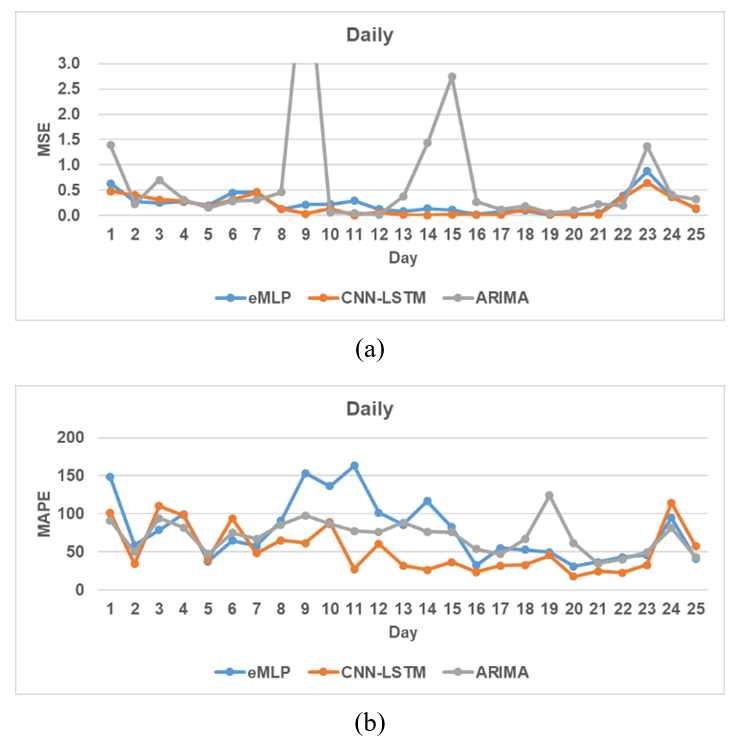### 4.2 Experimental Result

To evaluate accuracy, we should use the MSE and MAPE for electricity demand of the "kwh" level. Also, we used training data from between June 1, 2020, and June 30, 2021, and predicted electricity demand between July 1, 2021, and July 25, 2021. To compare whole models, we have predicted electricity demand in units of hours, and the corresponding results are shown in Table 2. Additionally, the results of MSE and MAPE for each day are shown in Fig. 7. Lastly, the performance comparison was done on Amazon Web Service (AWS) with Windows server 2016 x64, Intel Xeon Platinum 8259 CL CPU@2.50 GHz, 31.6GB RAM, and Python 3.7.8.

##### Fig. 8. Comparison of electricity demand accuracy between CNN-LSTM, eMLP, ARIMA, and the ground truth for 25 days.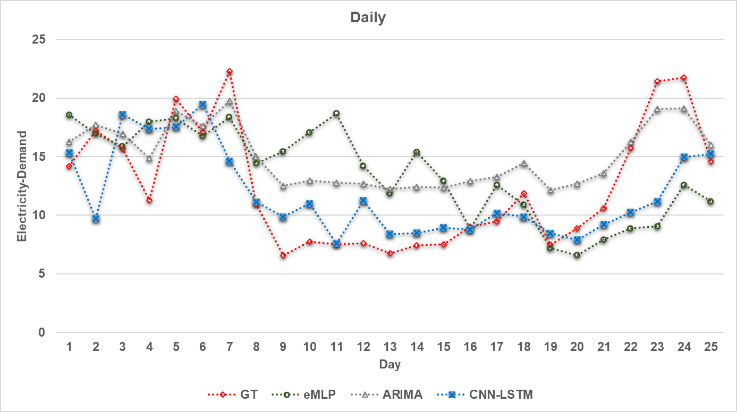##### Fig. 9. An example of comparison for electricity usage with prediction data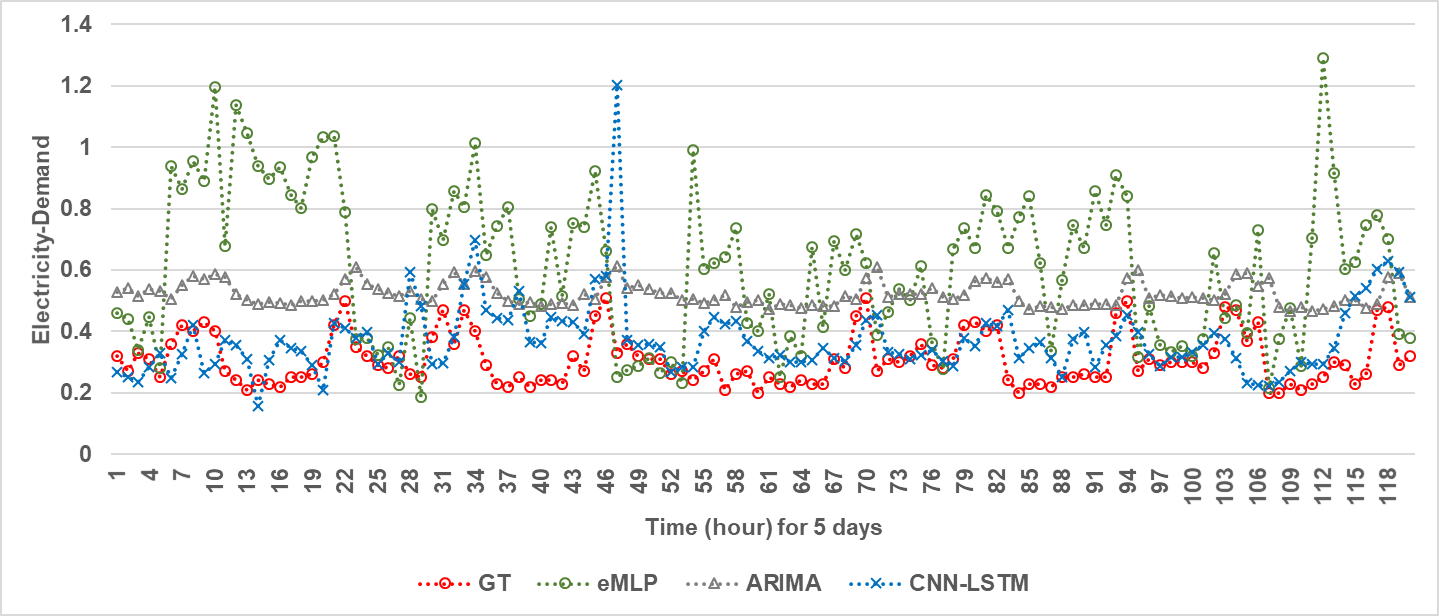## 5. Conclusion

Our main approach was electricity demand prediction and comparison with widely known ARIMA and deep-learning methods. MLP (eMLP) and LSTM with CNN (CNN-LSTM) were included to accomplish the tasks by deep learning. We focused on reaching good performance using the LSTM in time series analysis, and the most important factor for electricity demand prediction was weatherID due to processing by an embedding vector using the CNN. From the result, CNN-LSTM outperforms other methods in terms of MSE and MAPE, as shown in Fig. 8.

Overall, the CNN-LSTM model’s accuracy was just a little high for the irregular electricity demand dataset. Therefore, this model is also able to be applied to a PV prediction system [16-20] and electricity price prediction system [7, 27-29]. Overall, the electricity demand prediction system has an irregular pattern and has challenging tasks in terms of accuracy on the hour level. Therefore, it will be our future work to optimize the models.

### REFERENCES

1
Amarasinghe K., Marino D.L., Manic M., June 2017, Deep neural networks for energy load forecasting, in IEEE 26th International Symposium on Industrial Electronics2
Marino D.L., Amarasinghe K., Manic M., Oct. 2016, Building energy load forecasting using Deep Neural Networks, in IEEE Industrial Electronics Society3
Azadeh A., Faiz Z.S., 2011, A meta-heuristic framework for forecasting household electricity consumption, in Elsevier of Applied Soft Computing, Vol. 11, No. 1, pp. 614-6204
Mpawenimana I., Pegatoquet A., Roy V., Rodrguez L., Belleudy C., Oct. 2020, A comparative study of LSTM and ARIMA for energy load prediction with enhanced data preprocessing, in IEEE Sensors Applications Symposium5
Taylor J.W., McSharry P.E., Nov. 2007, Short-Term Load Forecasting Methods: An Evaluation Based on European Data, in IEEE Transactions on Power Systems, Vol. 226
Mpawenimana I., Pegatoquet A., Roy V., Rodriguez L., Belleudy C., 2020, A comparative study of LSTM and ARIMA for energy load prediction with enhanced data preprocessing, in IEEE Sensors Applications Symposium7
Tian H., Meng B., 2010, A new modeling method based on bagging ELM for day-ahead electricity price prediction, in IEEE International Conference on Bio-Inspired Computing8
Sheikhan M., Mohammadi N., 2012, Neural-based electricity load forecasting using hybrid of GA and ACO for feature selection, in Neural Computing and Applications 21, pp. 1961-19709
Fan L., Li J., Zhang X., Sep. 2020, Load Prediction Methods Using Machine Learning for Home Energy Management Systems Based on Human Behavior Patterns Recognition, in CSEE Journal of Power and Energy Systems, Vol. 6, No. 310
Taylor J.W., Menezes L.D , McSharry P.E., Jan.-Mar. 2006, A Comparison of univariate methods for forecasting electricity demand up to a day ahead, in International Journal of Forecasting, Vol. 22, No. 1, pp. 1-1612
Bedi J., Toshniwal D., 2019, Deep learning framework to forecast electicity demand, in Elsevier of Applied Energy, Vol. 238, pp. 1312-132613
Taylor J.W., 2003, Short-term electricity demand forecasting using double seasonal exponential smoothing, in Journal of the Operational Research Society, Vol. 54, No. 814
Mirasgedis S., Sarafidis Y., Georgopoulou E., Lalas D.P., Moschovits M., Karagiannis F., Papakonstantinou D., 2006, Models for mid-term electricity demand forecasting incorporating weather influences, in Elsevier of Energy, Vol. 31, No. 2-3, pp. pages 208-22715
Wijiaya T.K., Vasirani M., Humeau S., Aberer K., 2015, Cluster-based aggregate forecasting for residential electricity demand using smart meter data, in IEEE International Conference on Big Data 10.1109/BigData.2015.736383616
Almeida M.P., Perpinan O., Narvarte L., 2015, PV power forecast using a nonparametric PV model, in Elsevier of Solar Energy, Vol. 115, pp. 354-36817
Qamar M., Nadarajah M., Ekanayake C., 2016, On recent advances in PV output power forecast, in Elsevier of Solar Energy, Vol. 136, pp. 125-14418
Sanjari M.J., Gooi H.B., 2017, Probabilistic Forecast of PV Power Generation Based on Higher Order Markov Chain, in IEEE Transactions on Power Systems, Vol. 32, No. 419
Das U.K., Tey K.S., Seyedmahmoudian M., Idris M.Y.I., Mekhilef S., Horan B., Stojcevski A., 2017, SVR-Based Model to Forecast PV Power Generation under Different Weather Conditions, in MDPI of energies20
Meer D., Mouli G.R.C., Mouli G.M., Elizondo L.R., Bauer P., 2018, Energy Management System With PV Power Forecast to Optimally Charge EVs at the Workplace, in IEEE Transactions on Industrial Informatics, Vol. 14, No. 121
Zhang G.P., 2003, Time series forecasting using a hybrid ARIMA, neural network model, in Elsevier of Neurocomputing, Vol. 50, pp. 159-17522
Contreras J., Espinola R., Nogales F.J., Conejo A.J., 2003, ARIMA models to predict next-day electricity prices, in IEEE Transactions on Power Systems, Vol. 18, No. 323
Ho S.L., Xie M., 1998, The use of ARIMA models for reliability forecasting and analysis, in Elsevier of Computers & Industrial Engineering, Volume 35, Vol. 1, No. 2, pp. 213-21624
Chiu J.P.C., Nichols E., 2016, Named Entity Recognition with Bidirectional LSTM-CNNs, in Transactions of the Association for Computational Linguistics25
C.N. dos Santos , Guimaraes V., 2015, Boosting Named Entity Recognition with Neural Character Embeddings, in arXiv:1505.05008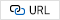26
Dietterich T.G., 2000, Ensemble Methods in Machine Learning, in International Workshop on Multiple Classifier Systems, pp. 1-1527
Peng L., Liu S., Liu R., Wang L., 2018, Effective long short-term memory with differential evolution algorithm for electricity price prediction, in Elsevier of Energy, Vol. 162, pp. 1201-131428
Chang Z., Zhang Y., Chen W., 2019, Electricity price prediction based on hybrid model of adam optimized LSTM neural network and wavelet transform, in Elsevier of Energy, Vol. 18729
Niimura T., Ko H.S., Ozawa K., 2002, A day-ahead electricity price prediction based on a fuzzy-neuro autoregressive model in a deregulated electricity market, in IEEE Proceedings of the International Joint Conference on Neural Networks## Author

Daegun Ko received his BSc in Electronic Engineering and Computer Engineering from Yeongnam University, South Korea, in 2009, and hold a Samsung Electronics Software Membership from 2006 to 2009. He received the MSc from the Department of Digital Media and Communications Engineering at Sungkyunkwan University, South Korea, in 2016. From 2009 to 2016, he was a research engineer at Samsung Electronics Co. Ltd., Suwon, South Korea, where he worked on optical character recognition, visual system, machine-learning and deep-learning. From 2016 to 2020, he was a research engineer at HP Inc., Pangyo, South Korea, where he worked on a lot of language modeling with letter recognition and natural language processing via deep-learning. Since January 2021, he has been with Hyundai-Autoever, Gangnam, South Korea. His research interests include image processing, pattern recognition, computer vision, time series prediction system, energy management system, optimal plan and natural language processing with deep-learning.

Youngmin Yoon received his B.E. in Electronic and Radio wave Engi-neering from Kyung Hee University, South Korea, in 2013. From 2013 to 2016, he was a software engineer at on Samsung Electronics Co. Ltd., Suwon, South Korea, where he worked on network firmware development. From 2016 to 2020, he was a research engineer at on HP Inc., Pangyo, South Korea, where he worked on optical character recognition. Since April 2021, he has been with Hyundai-Autoever, Gangnam, South Korea. His research interests include image processing, computer vision, time series forecasting system via deep-learning.

Jinoh Kim received his Bachelor’s degree in Multimedia Engineering from the Korea National Institute of Continuing Education in 2013. In 2019, he won the grand prize in the energy platform category at the High-tech Awards'' hosted by Korean company Hi-Tech information Co.,Ltd. He is currently the CEO of COREDIT, Inc. and also the Chief Architecture officer in the lab. His main technical work includes IT architecture consulting and systems engineering design. Since 2021, he has been studying for a PhD in Systems Engineering at Ajou University in South Korea. His research interests include how to efficiently configure and manage business platform architectures and how AI can be used to improve engineering processes.

Haelyong Choi received Ph.D. in Business Management at A Seoul School of Integrated Sciences & Technologies and working on Hyundai Autoever as Head of Sub-Division, Smartcity Service Group. He is performing various IT related projects of smartcity, energy, telecom, finance, and IoT platform since joining the company on August 16, 2006. His main research topics are regarding with B2B Sales, ICT, mechanism-based view and strategic product management fields.